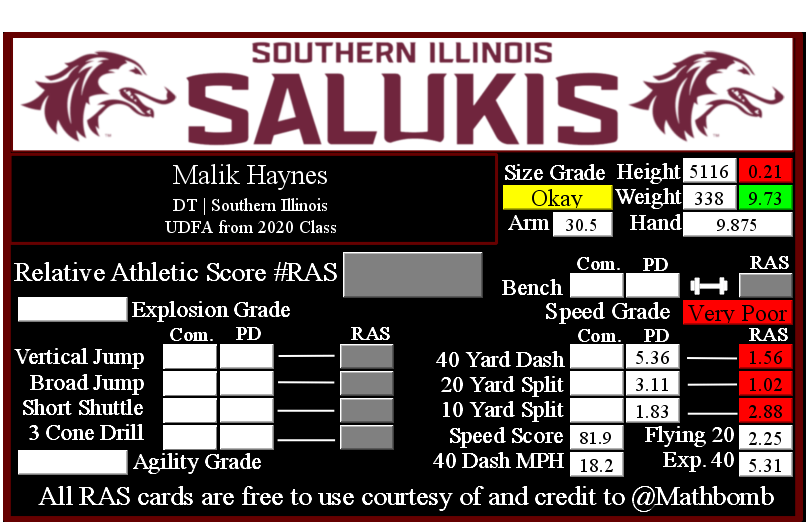# Malik Haynes RAS### Malik Haynes RASMalik Haynes went undrafted for the NFL out of Southern Illinois in 2020.

This player did not record a RAS either due to injury, choice, or due to metrics not being recorded.

He had a recorded height of 5116 that season, recorded as XYYZ where X is feet, YY is inches, and Z is eighths of an inch. That correlates to 5 feet, 11 and 6/8 of an inch or 71.75 inches, or 182.245 centimeters. This correlates to a 0.21 score out of 10.0.

He recorded a weight of 338 in pounds, which is approximately 153 kilograms. This correlates to a 9.73 score out of 10.0.

Based on his weight, he has a projected 40 yard dash time of 5.31. This is calculated by taking 0.00554 multiplied by his weight and then adding 3.433.

This player did not record any measurements at the NFL Combine that we were able to find.

At his pro day, he recorded a 40 yard dash of 5.36 seconds. This correlates to a 1.56 score out of 10.0.

The time traveled between the 20 and 40 yard lines is known as the Flying Twenty. As the distance is also known, we can calculate the player’s speed over that distance. The time he traveled the last twenty yards at his pro day was 2.25 seconds. Over 20 yards, we can calculate his speed in yards per second to 8.89. Taking into account the distance in feet (60 feet), we can calculate his speed in feet per second to 26.67. Breaking it down further, we can calculate his speed in inches per second to 320.0. Knowing the feet per second of 26.67, we can calculate the approximate miles per hour by multiplying that value by 0.681818 to give us a calculated MPH of 18.2 in the last 20 yards of his run.

At his pro day, he recorded a 20 yard split of 3.11 seconds. This correlates to a 1.02 score out of 10.0.

We can calculate the speed traveled over the second ten yards of the 40 yard dash easily, as the distance and time are both known. The time he traveled the second ten yards at his pro day was 1.28 seconds. Over 10 yards, we can calculate his speed in yards per second to 7.81. Taking into account the distance in feet (30 feet), we can calculate his speed in feet per second to 23.44. Breaking it down further, we can calculate his speed in inches per second to 281.25. Knowing the feet per second of 23.44, we can calculate the approximate miles per hour by multiplying that value by 0.681818 to give us a calculated MPH of 16.0 in the second ten yards of his run.

At his pro day, he recorded a 10 yard split of 1.83 seconds. This correlates to a 2.88 score out of 10.0.

The time he traveled the first ten yards at his pro day was 1.83 seconds. Over 10 yards, we can calculate his speed in yards per second to 5.0. Taking into account the distance in feet (30 feet), we can calculate his speed in feet per second to 16.0. Breaking it down further, we can calculate his speed in inches per second to 197.0. Knowing the feet per second of 16.0, we can calculate the approximate miles per hour by multiplying that value by 0.681818 to give us a calculated MPH of 10.9 in the first ten yards of his run.

This site uses Akismet to reduce spam. Learn how your comment data is processed.